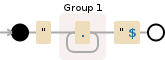# Regex - how to extract text from between quotes and exclude quotes

I need help with regex. I need to create a rule that would preserve everything between quotes and exclude quotes. For example: I want this...

``````STRING_ID#0="Stringtext";
``````

...turned into just...

``````Stringtext
``````

Thanks!

• More info needed: Can there be more than one quoted string in your input? Can there be escaped quotes? Which regex engine are you using? – Tim Pietzcker Nov 3 '11 at 16:16

The way to do this is with capturing groups. However, different languages handle capturing groups a little differently. Here's an example in Javascript:

``````var str = 'STRING_ID#0="Stringtext"';
var myRegexp = /"([^"]*)"/g;
var arr = [];

//Iterate through results of regex search
do {
var match = myRegexp.exec(str);
if (match != null)
{
//Each call to exec returns the next match as an array where index 1
//is the captured group if it exists and index 0 is the text matched
arr.push(match ? match : match);
}
} while (match != null);

document.write(arr.toString());
``````

The output is

``````Stringtext
``````
• You can do this shorter: let result = []; let regex = /myRegex/g; let match = regex.exec(data); while(match) { result.push(match); match = regex.exec(data); } – Kiechlus Apr 27 '16 at 12:43
``````"([^"\\]*(?:\\.[^"\\]*)*)"
``````

``````"(.+)"\$